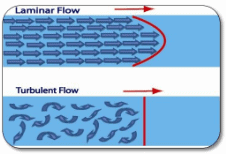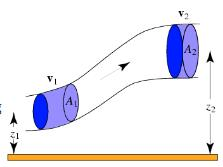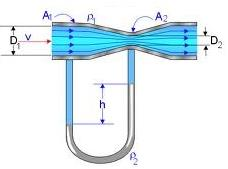Courses

# Notes | EduRev

## JEE Revision Notes

Created by: Learners Habitat

## JEE : Notes | EduRev

The document Notes | EduRev is a part of the JEE Course JEE Revision Notes.
All you need of JEE at this link: JEE

(Mechanical Properties of fluids):-

• Characteristic of Ideal fluid:-
(a) It is incompressible
(b) It is non-viscous
(c) Flow of ideal fluid is irrational
(d) It is capable of exhibiting steady flow
• Stream line flow:- Flow of a liquid fluid  is said to be streamlined if the velocity of a molecule, at any point, coincides with that of the preceding one.
• Tube of flow:- A bundle of streamlines having same velocity of fluid elements, over any cross-section perpendicular to the direction of flow, is called a tube of flow.
• Laminar flow:- It is a special case of streamline flow in which velocities of all the molecules on one streamline is same throughout its motion.
• Turbulent flow:- Whenever the velocity of a fluid is very high or it rushes past an obstacle so that there is a sudden change in its direction of motion, the motion of fluid becomes irregular, forming eddies or whirlpools. This type of motion of fluid is called turbulent flow.• Rate of flow (Equation of continuity):-av= Constant  (a1v1=a2v2)
Equation of continuity can be considered to be a statement of conservation of mass.
So, v ∝ 1/a
Velocity of flow of liquid varies inversely as the area of cross-section of the opening from where the liquid comes out.
• Total energy of a liquid:-
(a) Kinetic energy:- It is the energy possessed by a liquid by virtue of its velocity.
K.E = ½ mv2
K.E per unit mass = ½ v2
K.E per unit volume = ½ [mv2/V] = ½ ρv2
Here, ρ is the density of liquid.
(b) Potential energy:- It is the energy possessed by a liquid by virtue of which of its position.
Potential energy = mgh
P.E per unit mass = mgh/m = gh
P.E per unit volume = mgh/V = ρgh
(c)  Pressure energy:- It is the energy possessed by a liquid by virtue of its pressure.
Pressure energy = p×V = m (p/ρ)
Pressure energy per unit mass = p/ρ
Pressure energy per unit volume = p×V /V= p
• Total energy:- Total energy of a liquid is the sum total of kinetic energy, potential     energy and pressure energy.
E= ½ mv2 +mgh+mp/ρ
Total energy per unit mass = ½ v2 +gh+p/ρ
Total energy per unit volume = ½ ρv2 +ρgh+p
• Bernoulli’s equation:- It states that the total energy of a small amount of an incompressible non-viscous liquid flowing without friction from one point to another, in a streamlined flow, remains constant throughout the displacement.
(a) ½ mv2 + mgh+ mp/ρ = Constant
(b) ½ v2 +gh+p/ρ = Constant
(c) ½ ρv2 +ρgh+p = Constant   or  v2/2g + h + p/ρg = Constant
The term v2/2g is called velocity head, h is called gravitational head and p/ρg is called pressure head.
Therefore Bernoulli’s theorem states that in case of an incompressible, non-viscous fluid, flowing from one point to another in a streamlined flow, the sum total of velocity head, gravitational head and the pressure head is a constant quantity.
• Limitation of Bernoulli’s equation:-
(a) Force of viscosity, which comes into play in case of fluids in motion has not been accounted for.
(b) Loss of energy due to heat is not accounted for.
(c) When a fluid flows in a curved path, the energy due to centripetal force is also not accounted for.
• If v is the relative velocity of top layer w.r.t. any other deeper layer (may be the lowest), then v is lesser for greater depth.
v = K/bd
or v ∝ 1/d
• Venturimeter:-
It is a device used for measuring the rate of flow of liquids, generally water, through pipes.
The rate of flow of water, Q = a1a2√2hg/[a12-a22]• Torricelli’s theorem (velocity of efflux):-
It states that the velocity of efflux of a liquid (V), from an orifice, is equal to the velocity acquired by a body, falling freely (v), from the surface of liquid to the orifice.
So, V = v = √2gh
• Viscosity:- Viscosity is the property of fluids by virtue of which they tend to destroy any relative motion between their layers.
• Velocity gradient:- Velocity gradient is defined as the rate of change of velocity with respect to distance.
(b) Dimension of velocity gradient = [dv/dr] = [T-1]
(c) Direction of velocity gradient is perpendicular to the direction of flow, directed in the direction of increasing velocity.
(d) Average velocity gradient:- Average velocity gradient is the difference between velocities of two layers separated a unit distance apart.
• Newton’s law of viscosity:-
In accordance to Newton’s law of viscosity, the viscous drag force depends upon the nature of fluid along with following factors:-
(a) F∝A (common area of two layers)
(c) So, F =ηA (dv/dr)
Here η is called coefficient of viscosity of fluid.
• Coefficient of viscosity of fluid (ηv) or fugitive elasticity:-
ηv = shear stress/velocity gradient = (F/A)/(dv/dr)
• Modulus of rigidity(ηr):-
η= shear stress/shear strain = (F/A)/(θ) = (F/A)/(dx/dr)
Here, θ = dx/dr = displacement gradient
• Coefficient of viscosity (Absolute viscosity or Dynamic viscosity):-
F= ηA (dv/dr)    if A = 1, dv = 1, dr =1, F = η
Co-efficient of viscosity of a fluid is defined as the tangential force per unit area which is required to maintain (or resist) a unit relative velocity between two layers a unit distance apart.
Or
Co-efficient of viscosity of a fluid is defined as the tangential force per unit area which is required to maintain a unit velocity gradient between its layers.
Unit of η:-
S.I:- η = 1 deca poise = 1 N sec/m2
Co-efficient of viscosity of a fluid is said to be one deca-poise if a tangential force of 1 N per meter square is required to maintain a relative velocity of 1 ms-1 between its layer 1 m apart.
C.G.S:- η = 1 poise = 1 dyn sec/cm2
Coefficient of viscosity of a fluid is said to be one poise if a tangential force of 1 dyn per square cm is required to maintain a relative velocity of 1 cms-1 between its layers 1 cm apart.
• Relation between deca-poise and poise:-
1 deca-poise = 10 poise
• Dimension formula for η:-
• Fluidity:- Reciprocal of coefficient of viscosity of a fluid is called its fluidity.
Fluidity = 1/η
Unit of fluidity: poise-1
Dimension of fluidity: [M-1L1T1]
• Kinematic viscosity:- Kinematic viscosity of a fluid is defined as the ration between its coefficient of viscosity to the density of fluid.
Kinematic viscosity = η/ρ
Units of kinematic viscosity:- C.G.S – 1 stoke = cm2 s-1
Kinetic viscosity of a fluid having its dynamic viscosity one poise and density one g cm-3 is said to be 1 stoke.
Dimensional formula of kinematic viscosity = η/ρ = [M0L2T-1]
• Critical velocity (Reynold’s Number):- Critical velocity (vc) is the maximum velocity of the flow of liquid flowing in a streamlined flow.
v= NR η/ρD
Here η is the coefficient of viscosity of liquid, ρ is the density of liquid and D is the diameter of the tube.
Reynold’s Number, NR = ρvcD/ η
• Stokes law:- In accordance to Stoke’s law, force of viscosity F depend upon,
(a) Co-efficient of viscosity of fluid η
(b) Radius of the moving body r
(c) Velocity of body v
So, force of viscosity, F = 6π η r v
• Terminal velocity:- v = 2/9 [r2 (ρ-σ)/η]
• η = 2/9 [r2 (ρ-σ)g/v]
• Variation of viscosity with a change in temperature and pressure:-
(a) Effect of temperature:-
η= A /(1+Bt)c
Here A, B and C are constants.
Again, ηv1/2  = Aec/vt
Here, A and C are constants and v is the relative velocity.
(b) Effect of pressure:- Co-efficient of viscosity of liquids increases due to an increase in pressure but there is no relation, so far, to explain the effect.
• Change in viscosity of gases:-
(a) Effect of temperature:- Co-efficient of viscosity of a gas at a given temperature is given by,
η= η0AT1/2
Here T is the absolute temperature of gas.
Modified formula, η = [η0AT1/2]/[1+(S/T)]
(b) Effect of pressure:- At low pressure, co-efficient of viscosity of a gas varies directly with pressure.
• Rate of flow of liquid through a liquid through a capillary tube of radius r and length l,
V= πpr4/8ηl = p/(8ηl/ πr4) = p/R
Here p is the pressure difference between two ends of the capillary and R is the fluid resistance.
• Accelerated fluid containers:- tan θ = ax/g
• If W be the weight of a body and U be the up thrust force of the liquid on the body then,
(a) The body sinks in the liquid of W>U
(b) The body floats just completely immersed if W=U
• Pressure exerted by a column of liquid of height h:- P = hρg
Here, ρ is the density of liquid.
• Pressure at a point within the liquid:-
P = P0 +hρg
Here, P0 is the atmospheric pressure and h is the depth of point with respect to free surface of liquid.
Offer running on EduRev: Apply code STAYHOME200 to get INR 200 off on our premium plan EduRev Infinity!

233 docs

,

,

,

,

,

,

,

,

,

,

,

,

,

,

,

,

,

,

,

,

,

;